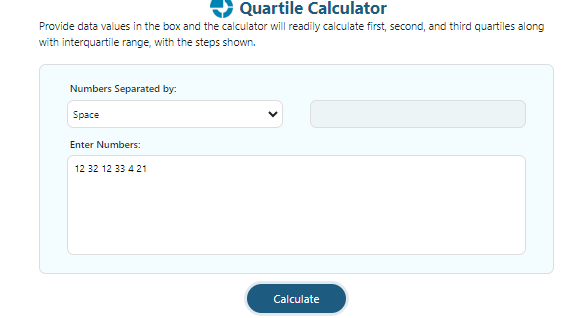# What Are Quartiles In Statistics & How To Calculate Them?

0

In statistics, the term quartile has a precise significance. And in the following article, we will discuss a couple of things that include:

• How to find quartiles
• How to use a free quartile calculator

So let’s move down to the article and start today’s concept!

## What Are Quartiles?

The particular values that help you to divide the large data set of numbers into quarters are known as the quartiles.

Usually, a quarter means four. It means that quartiles will equally divide a data set into four equal parts. By doing so, the task becomes very easy as you can easily and instantly simplify various statistical problems.

For example there are various quartiles of the profit margin, first identify the ROA with the assistance of an online ROA calculator developed by calculatored.com. Then know it quartile by inserting the values in the table.

And where you still face difficulty, let the lower quartile calculator by calculator-online.net does everything for you. Yes, this tool is specifically developed by calculator-online.net & helps you to calculate the quartiles of any small or large data.

## Quartile Formulas:

The quartile calculator uses the following formulas to calculate results without a delay and display them on your screen:

First Quartile(Q1) = ((n + 1)/4)th Term

Second Quartile(Q2) = ((n + 1)/2)th Term

Third Quartile(Q3) = (3(n + 1)/4)th Term

## How To Calculate First & Third Quartile?

Calculating quartiles becomes so fast and accurate if the procedure is done through the upper quartile calculator. But if your goal comes up with manual calculations, follow the points below

• Your data must be arranged from lowest to highest.
• You must now search for the median that will give the value for Q2
• You must divide the sorted data into two equal groups or halves at the median.
• The median of the lower half of the data is now Q1, while the median of the top half is Q3

## Example:

It is very easy to calculate Q1 and Q3. let us explore the following example!

### Statement:

Calculate the first and third quartiles for the following data set:

1, 4, 7, 5, 6, 4, 4, 7, 4, 8

#### Solution:

First of all, we have to arrange the data in ascending order as follows:

1, 4, 4, 4, 4, 5, 6, 7, 7, 8

Now if we look at the two center values which are 4 & 5, respectively. Calculating their average will give us the second quartile as follows:

Second quartile = 4 + 5 / 2

Second quartile = 9 / 2

Second quartile = 4.5

Which is the required second quartile that can also be verified by using the quartile calculator.

Now moving to the first and third quartiles. For this purpose, we have to split the data set into two equal parts:

First part: 1, 4, 4, 4, 4

Second part: 5, 6, 7, 7, 8

First quartile: 4 (Median of the first half)

Third quartile: 7 (Median of the second half)

## How To Use Quartile Calculator?

Using a calculator is quite straightforward! You need to follow these steps to use it effectively:• Select the way by which you will insert your data in the tool
• Enter the data set and hit Calculate
• You will get instant results with complete details

## Why Quartile Calculator?

• It is loaded with a simple user interface
• Quite fast results
• A lot of related parameters are determined
• You can use the tool without any limitations

## Things To Consider!

• A collection of observations is divided into four equal halves using the quartile formula. Between the first term and the median is where the first quartile is located
• The second quartile is the median
• The third quartile is the midpoint between the median and the last term

## Last Words:

In the following article, we briefly packed everything that is required to calculate quartiles. A large number of students and professionals use calculators to find quartiles due to their less result-oriented time. So to get instant results like them, you must start using the tool.

Tags